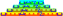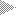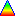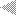# Script for printing a simple book cover

This is my "book-jacket" script. It is based on my own PS-generating Perl module, which is included below it. I am also using my own hacked-together GSF version of the TeX TT font, which is a bit lumpy but still a lot better than Courier or Courier-Bold, IMHO. See the very bottom for this.

Typical use: create a file called "out-jacket" in the current directory containing the spine text (first line) and front cover text (following lines). Run the script with something like "book-jacket 14", assuming the spine should be 14 mm wide. Alignment of the spine within the A4 piece of paper works for my HL-760 printer, but might need adjusting on other printers. (I did end up adjusting this script to send the page out upside down, because I found that that gave more reliable results.)

```
#!/usr/bin/perl

use JSP::TextPS;

sub usage {
die <<END;
Usage: book-jacket [options] <spine-thickness-mm>
-w  Double-width letters on spine
-ww Triple-width letters on spine (etc)
END
}

usage() unless (@ARGV);
\$mult= 1;
while (@ARGV > 0 && substr(\$ARGV, 0, 1) eq '-') {
my \$opt= substr(\$ARGV, 1);
shift @ARGV;
for (split(//, \$opt)) {
if (\$_ eq 'w') {
\$mult++;
}
else {
usage();
}
}
}

usage() unless (@ARGV);
\$spine= shift @ARGV;
\$spine *= 72 / 25.4;
\$spine= int(\$spine);

\$gap= 36;

\$z= new JSP::TextPS();
die "Can't find an `out-jacket' file"
unless open IN, "<out-jacket";

my @txt= ();
while (<IN>) {
s/^\s+//;
s/\s+\$//;
next if (\$_ eq '');
push @txt, \$_;
}
die unless close IN;

\$spmsg= shift @txt;

\$z->Grid(421 - \$gap, 595-\$gap, 180, 421-\$gap-\$gap, 595-\$gap-\$gap, 5, 0, 1, 1.0, "TeXTT", 0);
my \$rr= 5;
while (@txt) {
\$rr--; \$msg= pop @txt;
\$z->Text(\$rr, 0, \$msg);
}

(\$r, \$c, \$pt)= \$z->Fit(\$spine, 595, 1, 0, 0, 0.5);
\$c /= \$mult; 	# \$c= int(\$c);
\$z->Grid(421, 0, 0, \$spine, 595, 1, \$c, 0, \$mult, "TeXTT", 0);
\$z->Text(0, 0.5 * (\$c-length(\$spmsg)), \$spmsg);
#\$z->BBox(0, 0, 1, 1, 0);
#\$z->BBox(0, \$c-1, 1, 1, 0);

\$z->Output("out.ps");

print "See `out.ps'\n";

## END ##

```

This is my JSP::TextPS Perl module, required by the above script. It should be put somewhere like /usr/local/lib/site_perl/JSP/TextPS.pm (depending on your distribution):

```# Perl module for generating nice mono-spaced text PS files.
# Copyright (c) 2000-2003 Jim Peters <http://uazu.net/>.  This file is
# released under the same terms as Perl 5 itself.

package JSP::TextPS;
use strict;
use vars qw(@map8bit);

=pod

use JSP::TextPS;

\$xx= new JSP::TextPS(\$paptyp, \$land);
\$xx= new JSP::TextPS(\$paptyp);
\$xx= new JSP::TextPS();

Defaults to ('A4', 0) ie A4 portrait; \$land is non-0 for landscape
orientation.  \$paptyp may be [\$wid,\$hgt] for arbitrary sizes.

\$xx->TGray(\$lev);

Set default gray-level for text  (0: black/ink to 1: white/paper) (def 0)

\$xx->BGray(\$lev);

Set default gray-level for boxes (0: black/ink to 1: white/paper) (def 0)

\$xx->LWid(\$lwid);

Set default line-width for boxes (1.0: normal, <1.0 narrower etc.) (def 1)

(\$rr,\$cc,\$pt)= \$xx->Fit(\$hgt, \$wid, \$rows, \$cols, \$pt, \$rat);

Test to see how text will fit into a given rectangle, without using
condensing/leading.  Only one out of '\$rows', '\$cols' and '\$pt' should
be set; the other two should be zero.  The results are calculated
based on '\$rat', which is the width-to-point size ratio for the font:
0.6 for Courier, and 0.5 for TeXTT, and '\$hgt' and '\$wid' which are in
points.  Note that the results (esp '\$rr' and '\$cc') will not be
integers.

\$xx->Grid(\$tly, \$tlx, \$orient, \$hgt, \$wid,
\$rows, \$cols, \$lead, \$cond, \$font, \$pt);

Setup a grid to write characters/boxes onto.  Arguments are as
follows: (\$tly,\$tlx) top-left pos relative to top-left of paper, +ve y
is down, in points, (\$orient) orientation of grid relative to normal,
degrees, +ve anticlockwise rotation, (\$hgt,\$wid) height and width of
rectangle containing grid, in points, (\$rows,\$cols) number of rows and
columns of text to fit in rectangle, (\$lead) text leading factor: 1.0
normal, <1.0 less, >1.0 more leading, (\$cond) text condensing factor:
1.0 normal, <1.0 condensed, >1.0 expanded, (\$font) font name: eg
"Courier", or "TeXTT", (\$pt) point size.

Note that certain out of wid/hgt/cols/rows/cond/lead/pt may be 0, in
which case they are calculated from the other values.  In general one
of wid/cols/cond must be 0, and one of hgt/rows/lead, unless pt is 0,
in which case one set of 3 must be complete in order to calculate the
point size.

\$xx->GGrid(\$tly, \$tlx, \$orient, \$hgt, \$wid, \$rows, \$cols);
\$xx->GGrid(\$tly, \$tlx, \$orient, \$hgt, \$wid, \$rows);
\$xx->GGrid(\$tly, \$tlx, \$orient, \$hgt, \$wid);

Setup a grid that will be used for drawing boxes/circles only;
character cells are, by default, 1pt x 1pt, so '\$rows==\$hgt' and
'\$cols==\$wid'; rows and columns may be specified if required.

\$xx->Text(\$r, \$c, \$txt, \$gray, \$bhgt);
\$xx->Text(\$r, \$c, \$txt, \$gray);
\$xx->Text(\$r, \$c, \$txt);

Write text to the grid at (\$r,\$c).  '\$txt' may be a reference to an
array of strings (written line-by-line below the first), or a string
containing embedded newlines.  The gray-level may be specified if
required.  '\$bhgt' is the blank-line height, defaulting to 1.  Returns
the next row down available.  The lines may contain embedded
backspaces to overprint characters.  Characters 17->31 are mapped to
box parts: b3-b0: NSEW (see below).

\$xx->Box(\$r, \$c, \$h, \$w, \$gray, \$lwid);
\$xx->Box(\$r, \$c, \$h, \$w, \$gray);
\$xx->Box(\$r, \$c, \$h, \$w);

Draw a box with lines, between the centres of the characters in the
corners of the rectangle described.  Gray-level and line-width may be
specified.

\$xx->FBox(\$r, \$c, \$h, \$w, \$gray);
\$xx->FBox(\$r, \$c, \$h, \$w);

Draw a filled box between the centres of the characters in the corners
of the rectangle described.  Gray-level may be specified.

\$xx->BBox(\$r, \$c, \$h, \$w, \$gray);
\$xx->BBox(\$r, \$c, \$h, \$w);

Draw a filled background box covering the entire rectangle described.
Gray-level may be specified.

\$xx->Cir(\$r, \$c, \$h, \$w, \$gray, \$lwid);
\$xx->Cir(\$r, \$c, \$h, \$w, \$gray);
\$xx->Cir(\$r, \$c, \$h, \$w);

Draw an unfilled circle/ellipse touching the edges of the rectangle
specified.  Gray-level and line width may be specified.

\$xx->BCir(\$r, \$c, \$h, \$w, \$gray, \$rsz);
\$xx->BCir(\$r, \$c, \$h, \$w, \$gray);
\$xx->BCir(\$r, \$c, \$h, \$w);

Draw a filled circle/ellipse to fill the rectangle specified.
Gray-level may be specified.  '\$rsz' gives the relative size: 1=>100%
0.8=>80% etc.

\$xx->Line(\$r1, \$c1, \$r2, \$c2);

Draw a line from the top-left of one character to the top-left of
another.

\$xx->EndPage;

Mark the end of a page.

\$xx->Output(\$out);

Write the entire postscript file to a file named \$out.

\$xx->page_sx;

Page width (from parameters to TextPS->new()).

\$xx->page_sy;

Page height (from parameters to TextPS->new()).

\$txt= \$xx->Conv8bit(\$txt)

Convert 8-bit characters (accents etc) to overprinting PS sequences.

=over 4

=item * Note that row and column numbers start at (0,0) for top-left.
Fractional row/column numbers are permitted, and may be useful for
centering odd/even strings in table columns.

=item * Wherever a grey-level can be specified, an [r,g,b] array ref
may be used instead to specify a colour.

It is possible to temporarily set up a new grid within a region of the
current grid for drawing and/or text, and then to return to the
original grid once this is done.  This may be used for text effects
(bigger/smaller/stretched) within a layout.  Sub-grids may be nested.

\$xx->SubGrid(\$tly, \$tlx, \$orient, \$hgt, \$wid,
\$rows, \$cols, \$lead, \$cond, \$font, \$pt);

\$xx->SubGGrid(\$tly, \$tlx, \$orient, \$hgt, \$wid, \$rows, \$cols);
\$xx->SubGGrid(\$tly, \$tlx, \$orient, \$hgt, \$wid, \$rows);
\$xx->SubGGrid(\$tly, \$tlx, \$orient, \$hgt, \$wid);

Setup grids as for Grid() and GGrid().

\$xx->RestGrid;

Restore previous nested grid.

Box parts are represented by character codes 0x11 to 0x1F.  The low
nybble is shown below - imagine a leading 0x1 before each.

6--3--7--3--5         4		11 == 17   19 == 25
|     |     |         |		12 == 18   1A == 26
C     C     C         C		13 == 19   1B == 27
|     |     |         |		14 == 20   1C == 28
E--3--F--3--D   2--3--F--3--1	15 == 21   1D == 29
|     |     |         |		16 == 22   1E == 30
C     C     C         C		17 == 23   1F == 31
|     |     |         |		18 == 24
A--3--B--3--9         8

=cut

sub new {
my \$proto = shift;
my \$class = ref(\$proto) || \$proto;
my \$S  = {
plist => [],
curr => [],		# Current page (list of strings)
gray => "0 G",		# Initial gray-level
tgray => 0,		# Initial text gray-level
bgray => 0,		# Initial box gray-level
lwid => 1.0,		# Initial line width
sublev => 0		# Number of sub-grids active
};

my \$pgsize= shift || "A4";
my \$land= (@_) ? shift :  0;

# Fill in params
if ('ARRAY' eq ref \$pgsize) {
(\$S->{psx}, \$S->{psy})= @{\$pgsize};
\$S->{pname}= sprintf("%dx%d", @{\$pgsize});
} elsif ('A4' eq \$pgsize) {
\$S->{psx}= 595;
\$S->{psy}= 842;
\$S->{pname}= "A4";
} else {
die "Invalid page type: \$pgsize";
}

\$S->{landsc}= \$land;
if (\$land) {
\$S->{page_sx}= \$S->{psy};
\$S->{page_sy}= \$S->{psx};
} else {
\$S->{page_sx}= \$S->{psx};
\$S->{page_sy}= \$S->{psy};
}

bless (\$S, \$class);
return \$S;
}

sub TGray { my \$S= shift; \$S->{tgray}= shift; }
sub BGray { my \$S= shift; \$S->{bgray}= shift; }

sub LWid {
my (\$S, \$rat)= @_;
push @{\$S->{curr}}, "\$rat W"
unless (\$rat == \$S->{lwid});
\$S->{lwid}= \$rat;
}

sub EndPage {
my \$S= shift;
\$S->RestGrid while (\$S->{sublev});
push @{\$S->{plist}}, ["BEGIN", @{\$S->{curr}}, "END"];
\$S->{curr}= [];
}

sub set_gray {
my (\$S, \$lev)= @_;
\$lev= \$S->{bgray} if (\$lev == -1);
\$lev= \$S->{tgray} if (\$lev == -2);

if ('ARRAY' eq ref \$lev) {
my (\$r, \$g, \$b)= @{\$lev};
\$lev= (\$r << 16) + (\$g << 8) + \$b;
\$lev= "\$lev RGB";
} else {
\$lev= "\$lev G";
}

if (\$lev ne \$S->{gray}) {
\$S->{gray}= \$lev;
push @{\$S->{curr}}, \$lev;
}
}

sub Fit {
my (\$S, \$hgt, \$wid, \$rows, \$cols, \$pt, \$rat)= @_;
if (\$rows) {
\$pt= \$hgt / \$rows;
\$cols= \$wid / (\$pt * \$rat);
} elsif (\$cols) {
\$pt= \$wid / (\$cols * \$rat);
\$rows= \$hgt / \$pt;
} else {
\$rows= \$hgt / \$pt;
\$cols= \$wid / (\$pt * \$rat);
}
return (\$rows, \$cols, \$pt);
}

# Setup a grid to place text/boxes on; (tlx,tly) are relative to top-left of page,
#  in points, with +ve increasing down & right.  Takes care of landscape if set.
# Point size must be specified, or be derivable from either (wid,cols,cond) or
#  (hgt,rows,lead), in which case 0 is passed.  Once point size is established, one
#  out of (wid,cols,cond) must be 0, and one out of (hgt,rows,lead), which will be
#  calculated to fit the point size.

sub Grid {
my (\$S, \$tly, \$tlx, \$orient, \$hgt, \$wid, \$rows, \$cols, \$lead, \$cond, \$font, \$pt)= @_;

\$S->RestGrid while \$S->{sublev};
if (\$S->{landsc}) {
\$orient += 90;
(\$tly, \$tlx)= (\$tlx, \$tly);
} else {
\$tly= \$S->{psy} - \$tly;
}

die "Bad arguments to Grid - too many elements left as zero"
if (\$pt == 0 &&
!((\$wid && \$cols && \$cond) ||

push @{\$S->{curr}}, "\$orient \$tlx \$tly \$wid \$hgt \$cols \$rows \$cond \$lead \$pt /\$font TG";

# These are reset by 'TG'
\$S->{gray}= "0 G";
\$S->{lwid}= 1;
}

sub GGrid {
my (\$S, \$tly, \$tlx, \$orient, \$hgt, \$wid, \$rows, \$cols)= @_;
\$rows= \$hgt unless defined \$rows;
\$cols= \$wid unless defined \$cols;

\$S->RestGrid while \$S->{sublev};

if (\$S->{landsc}) {
\$orient += 90;
(\$tly, \$tlx)= (\$tlx, \$tly);
} else {
\$tly= \$S->{psy} - \$tly;
}

push @{\$S->{curr}}, "\$orient \$tlx \$tly \$wid \$hgt \$cols \$rows GG";

# These are reset by 'GG'
\$S->{gray}= "0 G";
\$S->{lwid}= 1;
}

sub SubGrid {
my (\$S, \$tly, \$tlx, \$orient, \$hgt, \$wid, \$rows, \$cols, \$lead, \$cond, \$font, \$pt)= @_;

die "Bad arguments to SubGrid - too many elements left as zero"
if (\$pt == 0 &&
!((\$wid && \$cols && \$cond) ||

push @{\$S->{curr}}, "\$orient \$tlx \$tly \$wid \$hgt \$cols \$rows \$cond \$lead \$pt /\$font STG";

# These are reset by 'STG'
\$S->{gray}= "0 G";
\$S->{lwid}= 1;
\$S->{sublev}++;
}

sub SubGGrid {
my (\$S, \$tly, \$tlx, \$orient, \$hgt, \$wid, \$rows, \$cols)= @_;
\$rows= \$hgt unless defined \$rows;
\$cols= \$wid unless defined \$cols;

\$S->RestGrid while \$S->{sublev};

push @{\$S->{curr}}, "\$orient \$tlx \$tly \$wid \$hgt \$cols \$rows SGG";

# These are reset by 'GG'
\$S->{gray}= "0 G";
\$S->{lwid}= 1;
\$S->{sublev}++;
}

sub RestGrid {
my \$S= shift;
push @{\$S->{curr}}, "RG";
die "Too many calls to RestGrid"
if (0 > --\$S->{sublev});
}

sub TextLine {
my (\$S, \$row, \$col, \$str, \$gray)= @_;
\$gray= -2 unless defined \$gray;

my \$col2= \$col;
my \$str2= \$str;

\$str =~ tr/\x11-\x1F/ /;		# Keep normal chars
\$str =~ s/([\(\)\\])/\\\$1/g;	# Quote ()\
\$str =~ s/\s+\$//;
\$str =~ s/^(\s*)//;
\$col += length(\$1);

if (\$str ne '') {
\$S->set_gray(\$gray);
push @{\$S->{curr}}, "(\$str) \$row \$col T";
}

\$str2 =~ tr/\x11-\x1F/ /c;		# Keep only box chars
\$str2 =~ tr/\x11-\x1F/A-Z/;		# Convert to A-O
\$str2 =~ s/\s+\$//;
\$str2 =~ s/^(\s*)//;
\$col2 += length(\$1);

if (\$str2 ne '') {
\$S->set_gray(\$gray);
push @{\$S->{curr}}, "(\$str2) \$row \$col2 BT";
}
}

# Write a block of text; 'str' may be a string containing newline
# characters, or a reference to an array of strings.  The lines may
# contain BS (0x08) characters to overprint characters.  Box
# characters are 0x11 to 0x1F.

sub Text {
my (\$S, \$row, \$col0, \$str, \$gray, \$bhgt)= @_;
\$gray= -2 unless defined \$gray;
\$bhgt= 1 unless defined \$bhgt;

for (('ARRAY' eq ref \$str) ? @{\$str} : split(/\n/, \$str)) {
if (/^\s*\$/) {
\$row += \$bhgt;
next;
}
my \$col= \$col0;
for my \$seg (split(/\010/, \$_)) {
\$S->TextLine(\$row, \$col, \$seg, \$gray);
\$col += length(\$seg) - 1;
}
\$row++;
}
return \$row;
}

sub Box {
my (\$S, \$row, \$col, \$hgt, \$wid, \$gray, \$lwid)= @_;
\$lwid= 1 unless defined \$lwid;

\$S->set_gray(defined(\$gray) ? \$gray : -1);
\$S->LWid(\$lwid);
push @{\$S->{curr}}, "\$row \$col \$hgt \$wid B";
}

sub FBox {
my (\$S, \$row, \$col, \$hgt, \$wid, \$gray)= @_;
\$S->set_gray(defined(\$gray) ? \$gray : -1);
push @{\$S->{curr}}, "\$row \$col \$hgt \$wid FB";
}

sub BBox {
my (\$S, \$row, \$col, \$hgt, \$wid, \$gray)= @_;
\$S->set_gray(defined(\$gray) ? \$gray : -1);
push @{\$S->{curr}}, "\$row \$col \$hgt \$wid BB";
}

sub Cir {
my (\$S, \$row, \$col, \$hgt, \$wid, \$gray)= @_;
\$S->set_gray(defined(\$gray) ? \$gray : -1);
push @{\$S->{curr}}, "\$row \$col \$hgt \$wid C";
}

sub BCir {
my (\$S, \$row, \$col, \$hgt, \$wid, \$gray, \$rsz)= @_;
\$rsz= 1 unless defined \$rsz;
\$S->set_gray(defined(\$gray) ? \$gray : -1);
push @{\$S->{curr}}, "\$row \$col \$hgt \$wid \$rsz BC";
}

sub Line {
my (\$S, \$r1, \$c1, \$r2, \$c2, \$gray)= @_;
\$S->set_gray(defined(\$gray) ? \$gray : -1);
push @{\$S->{curr}}, "\$r1 \$c1 \$r2 \$c2 BC";
}

sub Output {
my (\$S, \$fnam)= @_;
\$S->EndPage if (@{\$S->{curr}});
die "Can't create output file: \$fnam"
unless open TEXTPS_OUT, ">\$fnam";
my \$n_pages= @{\$S->{plist}};
print STDERR "Pages: \$n_pages\n";

my @tmp;

push @tmp, "%%Pages: \$n_pages";
push @tmp, "%%BoundingBox: 0 0 \$S->{psx} \$S->{psy}";
push @tmp, "%%Orientation: " . (\$S->{landsc} ? "Landscape" : "Portrait");
push @tmp, "%%DocumentMedia: \$S->{pname} \$S->{psx} \$S->{psy} 75 white ()";
push @tmp, <<'END-PS';
%%BeginProlog
/WorkDict 20 dict def
WorkDict begin
/xdef { exch def } bind def
/abort { /_aborting_ add } bind def
/BEGIN { gsave } bind def
/END { grestore showpage } bind def
/G { setgray } bind def
/RGB { dup -16 bitshift 255 and 255 div exch
dup -8 bitshift 255 and 255 div exch
255 and 255 div setrgbcolor } bind def
/W { mx 0.2 mul mul setlinewidth } def
/chkzeros {0 3 {exch 0 eq {1 add} if} repeat dup 2 ge {abort} if 1 eq} bind def
/TG {
grestore gsave
findfont /f xdef /pt xdef /lead xdef /cond xdef
/rr xdef /cc xdef /sy xdef /sx xdef
translate rotate
/csx f setfont (O) stringwidth pop def
/zyy lead rr sy chkzeros def
/zxx cond cc sx chkzeros def
pt 0 eq {
zxx {zyy {abort} {sy rr div lead div /pt xdef} ifelse }
{sx cc div cond div csx div /pt xdef} ifelse } if
zxx {
cond 0 eq {/cond sx cc pt csx mul mul div def} if
cc 0 eq {/cc sx pt csx cond mul mul div def} if
sx 0 eq {/sx cc pt csx cond mul mul mul def} if } if
zyy {
rr 0 eq {/rr sy pt lead mul div def} if
sy 0 eq {/sy rr pt lead mul mul def} if } if
0 0 moveto sx 0 lineto sx sy neg lineto 0 sy neg lineto closepath clip newpath
f pt scalefont setfont
0 0 moveto (O) false charpath flattenpath pathbbox newpath
cond 1 scale
/mx pt csx mul def /my pt lead mul neg def
0 G 1 W
} def
/GG {
grestore gsave
/rr xdef /cc xdef /sy xdef /sx xdef
translate rotate
0 0 moveto sx 0 lineto sx sy neg lineto 0 sy neg lineto closepath clip newpath
0 /cadj xdef 1 /cond xdef
/mx sx cc div def /my sy rr div neg def
my 0.5 mul 0 exch translate
0 G 1 W
} def
/STG {
1 cond div 1 scale
gsave
f pt lead cond rr cc sy sx cadj mx my 22 11 roll
11 6 roll
my mul neg 11 1 roll
mx cond mul mul 11 1 roll
mx cond mul mul 11 2 roll
TG
} def
/SGG {
1 cond div 1 scale
gsave
f pt lead cond rr cc sy sx cadj mx my 18 11 roll
7 2 roll
my mul neg 7 1 roll
mx cond mul mul 7 1 roll
mx cond mul mul 7 2 roll
GG
} def
/RG {
grestore
/my xdef /mx xdef /cadj xdef /sx xdef /sy xdef
/cc xdef /rr xdef /cond xdef /lead xdef /pt xdef
/f xdef
cond 1 scale
} def

/T { mx mul exch my mul moveto show } def
2 index 1 and 0 ne {mx -0.5 mul 0 rlineto 2 copy lineto} if
2 index 2 and 0 ne {mx 0.5 mul 0 rlineto 2 copy lineto} if
2 index 4 and 0 ne {0 my 0.5 mul rlineto 2 copy lineto} if
2 index 8 and 0 ne {0 my -0.5 mul rlineto 2 copy lineto} if
pop pop pop stroke } def
/BT { 3 2 roll {3 copy pop BP 1 add} forall pop pop } def
ww 0 rlineto 0 hh rlineto ww neg 0 rlineto closepath stroke } def
ww 0 rlineto 0 hh rlineto ww neg 0 rlineto closepath fill } def
/BB { 4 2 roll mx mul exch -0.5 add my mul cadj add moveto mx mul /ww xdef my mul /hh xdef
ww 0 rlineto 0 hh rlineto ww neg 0 rlineto closepath fill } def
/C { 4 2 roll mx mul /xx xdef -0.5 add my mul cadj add /yy xdef mx mul /ww xdef my mul /hh xdef
gsave [ 1 0 0 hh ww div xx yy ] concat ww 0.5 mul dup dup 360 0 arcn closepath stroke grestore } def
/BC { 0.5 mul /rsz xdef
4 2 roll mx mul /xx xdef -0.5 add my mul cadj add /yy xdef mx mul /ww xdef my mul /hh xdef
gsave [ ww 0 0 hh xx yy ] concat 0.5 0.5 rsz 360 0 arcn closepath fill grestore } def
end
%%EndProlog
%%BeginSetup
WorkDict begin
END-PS

push @tmp, "%%PaperSize: \$S->{pname}";
push @tmp, "%%EndSetup\n";
print TEXTPS_OUT join("\n", @tmp);

my \$pnum= 0;
for my \$pag (@{\$S->{plist}}) {
\$pnum++;
print TEXTPS_OUT "%%Page: \$pnum \$pnum\n";

my \$ind= 0;
for (@{\$pag}) {
my \$len= length(\$_);
if (\$ind + \$len > 75) {
print TEXTPS_OUT "\n", \$_;
\$ind= \$len;
} else {
print TEXTPS_OUT " ", \$_;
\$ind += \$len + 1;
}
}
print TEXTPS_OUT "\n" if (\$ind);
}

print TEXTPS_OUT "%%Trailer\n";
print TEXTPS_OUT "end\n";
print TEXTPS_OUT "%%EOF\n";
die "Error writing to \$fnam" unless close TEXTPS_OUT;

# Release memory consumed by stored pages
\$S->{plist}= [];
\$S->{curr}= [];
}

sub page_sx { my \$S= shift; return \$S->{page_sx}; }
sub page_sy { my \$S= shift; return \$S->{page_sy}; }

@map8bit= ("<\x08>", "\xA1", "\xA2", "\xA3", "<\x08>", "\xA5", "<\x08>", "<\x08>",
"<\x08>", "<\x08>", "<\x08>", "<\x08>", "<\x08>", "<\x08>", "<\x08>", "<\x08>",
"\xCA", "<\x08>", "\xC5", "<\x08>", "<\x08>", "<\x08>", "<\x08>", "\xCF",
"<\x08>", "<\x08>", "<\x08>", "<\x08>", "<\x08>", "<\x08>", "<\x08>", "\xBF",
"<\x08>", "<\x08>", "<\x08>", "<\x08>", "A\x08\xC8", "<\x08>", "X", "C\x08\xCB",
"<\x08>", "E\x08\xC2", "<\x08>", "<\x08>", "<\x08>", "<\x08>", "<\x08>", "<\x08>",
"<\x08>", "N\x08\xC4", "<\x08>", "<\x08>", "<\x08>", "<\x08>", "O\x08\xC8", "<\x08>",
"<\x08>", "<\x08>", "<\x08>", "<\x08>", "U\x08\xC8", "<\x08>", "<\x08>", "<\x08>",
"a\x08\xC1", "a\x08\xC2", "a\x08\xC3", "<\x08>", "a\x08\xC8", "<\x08>", "<\x08>", "c\x08\xCB",
"e\x08\xC1", "e\x08\xC2", "e\x08\xC3", "e\x08\xC8", "<\x08>", "i\x08\xC2", "i\x08\xC3", "i\x08\xC8",
"<\x08>", "n\x08\xC4", "<\x08>", "o\x08\xC2", "o\x08\xC3", "<\x08>", "o\x08\xC8", "<\x08>",
"<\x08>", "<\x08>", "u\x08\xC2", "u\x08\xC3", "u\x08\xC8", "<\x08>", "<\x08>", "<\x08>"
);

sub Conv8bit {
my (\$S, \$str)= @_;
\$str =~ s/([\xA0-\xFF])/\$map8bit[ord(\$1)-160]/eg;
return \$str;
}

1;

## END ##
```

The final bit you require to reproduce my setup exactly (assuming this is really what you want to do) is to install my TeXTT GhostScript font. This is a crude translation of the TeX typewriter monospace font into a GhostScript font. You need to add the following line to your /etc/gs.Fontmap (for Debian at least):

/TeXTT (tt10.gsf) ;These pages and files, including applets and artwork, are Copyright (c) 1997-2019 Jim Peters unless otherwise stated. Please contact me if you'd like to use anything not explicitly released, or if you have something interesting to discuss.